/

### The comonotonicity copula

The Comonotonicity copula is a special copula characterising perfect positive dependence, in the sense that the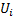are almost surely strictly increasing functions of each other.

 Copula name comonotonicity copula Common notation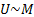Parameters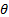Domain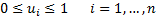Copula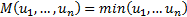Kendall’s rank correlation coefficient (for bivariate case)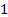Coefficient of upper tail dependenceCoefficient of lower tail dependenceOther comments Is the extreme case where dependency is as strongly “positive” as possible (i.e. achieves the Fréchet upper bound)

Nematrian web functions

Functions relating to the above distribution may be accessed via the Nematrian web function library by using a DistributionName of “Comonotonicity Copula”. For details of other supported probability distributions see here.